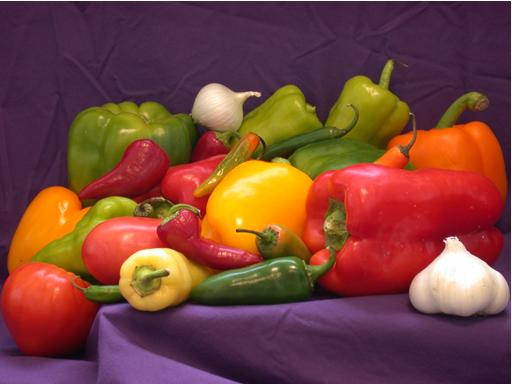# Counting occurrences of image colors

Blog reader André recently asked me how to count the number of times each color appears in an image. One short solution involves optional output arguments from the function unique, as well as the relatively new function accumarray.

Let's look first at the function unique. Probably most people use it with a single output argument, like this:

v = [1 1 3 5 5 2 3 2 2]
v =

1     1     3     5     5     2     3     2     2


u = unique(v)
u =

1     2     3     5



unique can also return two additional outputs:

[u, m, n] = unique(v);
m
m =

2     9     7     5


n
n =

1     1     3     4     4     2     3     2     2



So what do m and n mean? Well, m tells you where you can find the unique values in the original vector. Specifically, m(1) is the index of the last occurrence of the value u(1) in the original vector v. Similarly, m(3) is the index of the last occurrent of the value u(3) in v.

fprintf('m(3) = %d; u(3) = %d, v(m(3)) = %d\n', m(3), u(3), ...
v(m(3)))
m(3) = 7; u(3) = 3, v(m(3)) = 3


The output argument n gives you indices you can use to reconstruct the original vector from the vector of unique values. In orders, v equals u(n).

u(n)
ans =

1     1     3     5     5     2     3     2     2



If you count how many times the value 2 appears in the vector n, that will tell you how many times the value u(2) appears in the vector v. That brings us to the second function I mentioned, accumarray. Here's the pertinent sentence from the function description in the doc:

A = accumarray(subs, val) creates an array A by accumulating elements of the vector val using the subscript in subs.

Passing n as subs and 1 as val to accumarray gives us a way to count how many times each value of n appears.

counts = accumarray(n(:), 1)
counts =

2
3
2
2



counts(1) is the number of times that the value u(1) appears in v. counts(2) is the number of times that the value u(2) appears in v, and so on.

for k = 1:numel(counts)
fprintf('The value %d appears %d times in v.\n', ...
u(k), counts(k));
end
The value 1 appears 2 times in v.
The value 2 appears 3 times in v.
The value 3 appears 2 times in v.
The value 5 appears 2 times in v.


So unique and accumarray help to count the number of occurrences of scalars. How do we adapt this technique to count colors? Specifically, how do we count how many times each unique color occurs in a M-by-N-by-3 truecolor image?

First, we reshape the image so that the columns correspond to three color channels.

rgb = imread('peppers.png');
imshow(rgb)size(rgb)
ans =

384   512     3


rgb_columns = reshape(rgb, [], 3);
size(rgb_columns)
ans =

196608           3



Then we use our procedure above with unique and accumarray, except that the we add the 'rows' option to unique.

[unique_colors, m, n] = unique(rgb_columns, 'rows');
color_counts = accumarray(n, 1);

fprintf('There are %d unique colors in the image.\n', ...
size(unique_colors, 1))
There are 99059 unique colors in the image.


Finally, let's find out what color occurs most frequently.

[max_count, idx] = max(color_counts);

fprintf('The color [%d %d %d] occurs %d times.\n', ...
unique_colors(idx, 1), unique_colors(idx, 2), ...
unique_colors(idx, 3), max_count)
The color [254 254 254] occurs 344 times.


And where does this common color occur?

bw = n == idx;
bw = reshape(bw, size(rgb, 1), size(rgb, 2));
imshow(bw)Published with MATLAB® 7.5

|